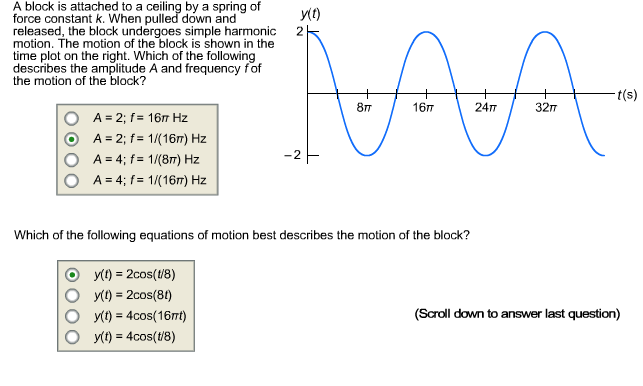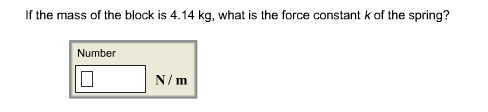# A block is attached to a ceiling by a spring ofy(1)force constant k. When pulled down andreleased, the block undergoes simple harmonicmotion. The motion of the block is shown in thetime plot on the right. Which of the followingdescribes the amplitude A and frequency fofthe motion of the block?2t(s)16п24T32п8тA = 2; f= 16 HzA = 2; f= 1/(16m) HzA = 4; f = 1/(8m) HzA = 4; f= 1/(16m) HzWhich of the following equations of motion best describes the motion of the block?y(1) = 2cos(18)y(1) = 2cos(81)y(t) = 4cos(16nt)y(t) = 4cos(t/8)%3D(Scroll down to answer last question) If the mass of the block is 4.14 kg, what is the force constant k of the spring?NumberN/m

Question
25 viewshelp_outlineImage TranscriptioncloseA block is attached to a ceiling by a spring of y(1) force constant k. When pulled down and released, the block undergoes simple harmonic motion. The motion of the block is shown in the time plot on the right. Which of the following describes the amplitude A and frequency fof the motion of the block? 2 t(s) 16п 24T 32п 8т A = 2; f= 16 Hz A = 2; f= 1/(16m) Hz A = 4; f = 1/(8m) Hz A = 4; f= 1/(16m) Hz Which of the following equations of motion best describes the motion of the block? y(1) = 2cos(18) y(1) = 2cos(81) y(t) = 4cos(16nt) y(t) = 4cos(t/8) %3D (Scroll down to answer last question) fullscreenhelp_outlineImage TranscriptioncloseIf the mass of the block is 4.14 kg, what is the force constant k of the spring? Number N/m fullscreen
check_circle

star
star
star
star
star
1 Rating
Step 1

a)

Refer given figure, the amplitude and frequency is,

Step 2

Hence option (2) is correct.

b)

The expression ...

### Want to see the full answer?

See Solution

#### Want to see this answer and more?

Solutions are written by subject experts who are available 24/7. Questions are typically answered within 1 hour.*

See Solution
*Response times may vary by subject and question.
Tagged in

### Mechanical Properties of Matter